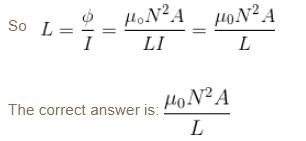Courses

# Faraday's Law MCQ Level - 1

## 10 Questions MCQ Test Topic wise Tests for IIT JAM Physics | Faraday's Law MCQ Level - 1

Description
This mock test of Faraday's Law MCQ Level - 1 for Physics helps you for every Physics entrance exam. This contains 10 Multiple Choice Questions for Physics Faraday's Law MCQ Level - 1 (mcq) to study with solutions a complete question bank. The solved questions answers in this Faraday's Law MCQ Level - 1 quiz give you a good mix of easy questions and tough questions. Physics students definitely take this Faraday's Law MCQ Level - 1 exercise for a better result in the exam. You can find other Faraday's Law MCQ Level - 1 extra questions, long questions & short questions for Physics on EduRev as well by searching above.
QUESTION: 1

### The induced emf in a coil rotating in a uniform magnetic field depend upon.

Solution:

The correct answer is: All of the above

QUESTION: 2

Solution:

QUESTION: 3

### A conducting rod is moved with a constant velocity v in a magnetic field. A potential difference appears across the two ends.

Solution:

The correct answer is: None of these

QUESTION: 4

A metallic rod completes its circuit as shown in the figure. The circuit is normal to a magnetic field of B = 0.15 tesla. If the resistances of the rod is 3Ω  the force required to move the rod with a constant velocity of 2 m/sec is -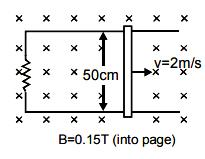Solution: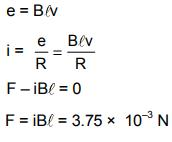QUESTION: 5

A coil of metal wire is kept stationary in a uniform magnetic field :

Solution:

If the coil is kept stationary in the uniform magnetic field, there will be no change in the magnetic flux linked with the coil. Therefore, there will be no induced emf in the coil.

Since, there is no induced emf, there will be no induced current also in the coil.

QUESTION: 6

The magnetic flux φ (in  weber) in a closed circuit of resistance 10Ω varies with time t (in second) according to equation φB = 6t2 - 5t + 1. The magnitude of induced current at t = 0.25sec  is :

Solution:

If the flux of magnetic field across the coil varies with time an emf is induced in it.

The induced emf is equal to negative rate of change of magnetic flux across it.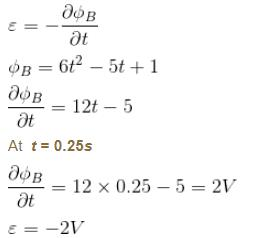Magnitude of induced emf = 2V, magnitude of induced current  i = 0.2 A

QUESTION: 7

A coil has an area of 0.05 m2 and it has 800 turns. It is placed perpendicularly in a magnetic field of strength 4 × 10–5 wb/m2. It is rotated through 90° in 0.1s. The average emf induced in the coil is :

Solution:

Induced emf,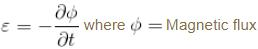Where flux through the coil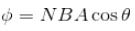θ is the angle between magnetic field and unit normal to the surface.

when coil is rotated from 0° to 90° then total change in magnetic flux is given by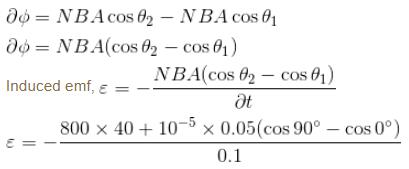0.016 V
The correct answer is: 0.016 V

QUESTION: 8

A magnet is brought towards a coil (i) speedily and (ii) slowly then the induced emf/induced charge will be respectively.

Solution: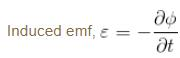The magnitude of induced emf is directly proportional to the rate of change of magnetic flux. So induced emf will be more in first case.

We also know ε = iR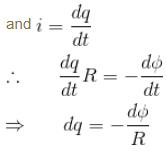So, induced charge in a coil is independent of time taken to charge the flux.
The correct answer is: more in first case/equal in both case

QUESTION: 9

The current carrying wire and rod AB are in the same plane. The rod moves parallel to the wire with a velocity v. Which one of the following statement is true about induced emf in the rod.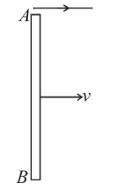Solution:

When the rod move in the magnetic field due to current carrying wire. The emf is induced in the rod.

Magnetic field due to current carrying wire is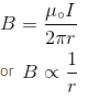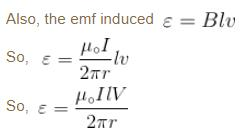So  B is farther from  A
So  B is at lower potential.
The correct answer is: Potential at A  will be higher than at  B

QUESTION: 10

The self inductance of solenoid of length L area of cross-section A and having N turns is

Solution:

Let a solenoid cross-sectional area A and N  turns. We know, in case of solenoid magnetic field along the axis of solenoid is given by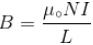Where N  is the number of turns and I  is the current flowing in the solenoid.
Flux through coil of solenoid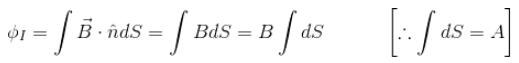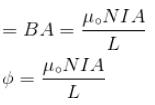Total flux = number of turns × flux through one coil.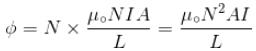Now we know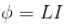where L  is self inductance